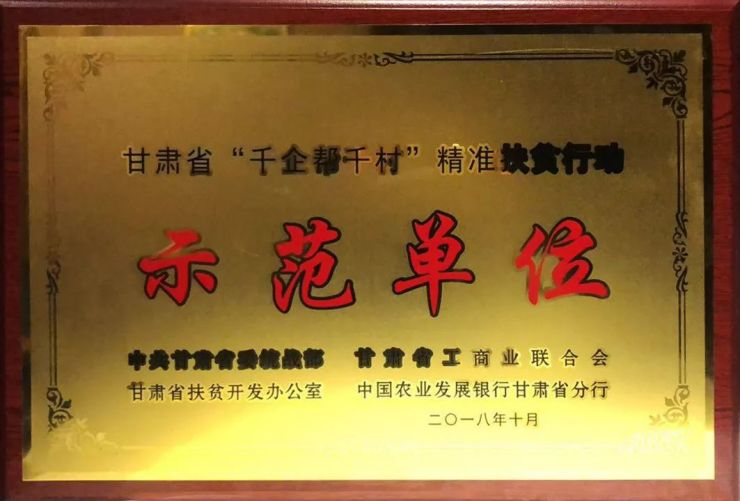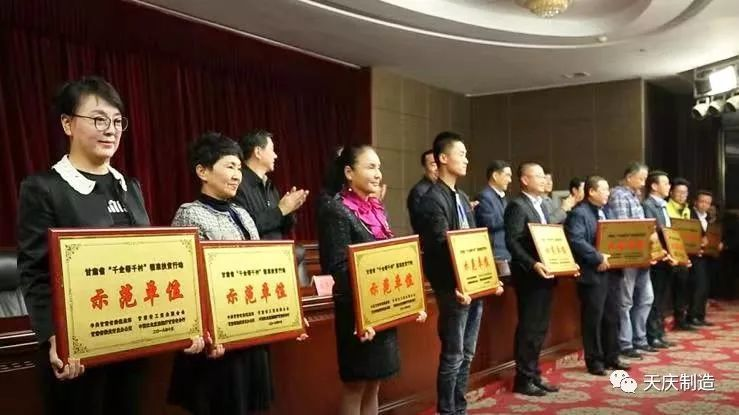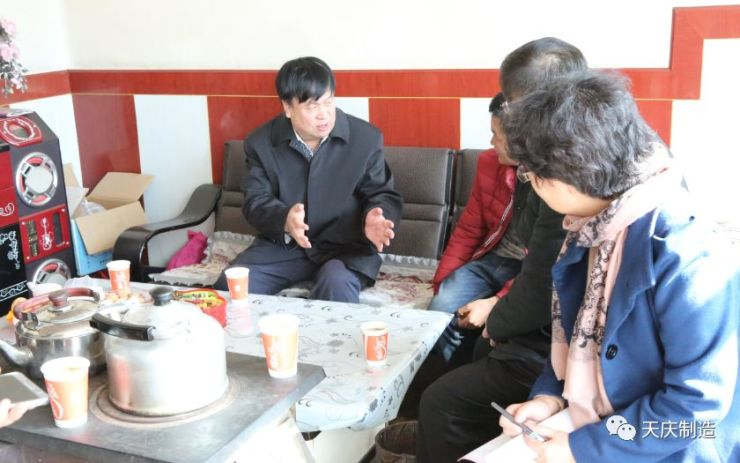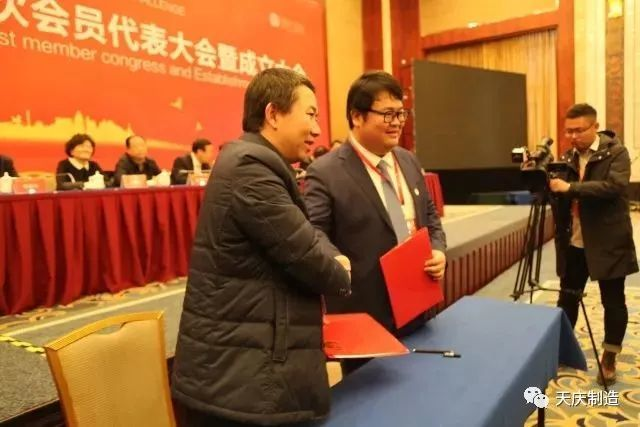|

# 头条|天庆集团获甘肃省首批“千企帮千村”精准扶贫行动示范单位荣誉

10月18日，在第5个全国“扶贫日”次日，甘肃省工商联在陇南市召开甘肃省“千企帮千村”工作会推进会暨民营企业与陇南市农民合作专业社对接会，动员非公经济人士积极参与到脱贫攻坚行动中来，会议还对天庆集团等第一批“千企帮千村”示范单位进行授牌。牢记嘱托，感恩奋进。天庆集团在企业发展壮大的同时，始终不忘深入贯彻国家精准扶贫、精准脱贫基本方略。十八年来，累计捐资捐助1亿余元用于精准扶贫、教育扶贫、抗震救灾、扶贫济困、关爱儿童和孤寡老人等慈善事业中，践行着“努力做最受尊敬的企业”愿景和“共同富裕”的时代呼唤。教育+产业 扶贫模式与时俱进

实现精准脱贫，造血帮扶是核心。天庆集团积极响应省工商联“千企帮千村”和省、市两级精准扶贫行动要求，对口帮扶合作市卡加曼乡香拉村，转“输血”为“造血”，出资150万元，购买奶牛150头，为香拉村修建奶牛养殖场，并以奶牛场运营为依托，设立“共济帮扶基金”，基金全部用于村辖141名农户，真正使扶贫利益共享、全员参与分配。目前，奶牛场存栏奶牛已达210余头，被评定为“甘南州标准化奶牛养殖场”。截止2017年，村民人均年收入增加到两万余元，为香拉村经济发展和扶贫开发工作起到了积极的作用。“扶贫先扶智”，教育扶贫是最根本的精准扶贫方式。十八年来，天庆集团在省委统战部、省、市工商联和各级政府及相关部门的领导下，帮扶兰州市贫困家庭及五保户，共计200余户近千人，助其渡过人生难关，通过对帮扶对象的持续回访，不断总结探索帮扶新模式。自2007年起，天庆集团以培育一个大学生，给家庭点燃希望之火为着眼点，在“扶贫”的基础上更加注重“扶智”，增强“造血”功能塑造，连续12年开展“送天庆助学情 圆莘莘学子梦”系列助学活动，累计帮扶3000多名困难大学生完成学业，发放助学款4000余万元。同时投资近千万，援建五所天庆慈爱小学，自建一所中学，一所小学，十所幼儿园，从“根子”上脱贫出实策，办实事。2018年天庆集团再捐260万元用于教育扶贫，12年来已累计资助3000余名学子

国务院扶贫办相关领导在调研中表示，天庆集团拿出真金白银、真情实干、真抓实干，实施“送天庆助学情 圆莘莘学子梦”活动，以教育脱贫拔穷根，产业扶贫项目“天庆香拉奶牛养殖场”成效显著，天庆集团探索出的教育+产业的精准帮扶模式，充分考虑了贫困地区的地理、人文等特点，不仅改变了贫穷家庭的面貌，更通过市场化手段有机连接，建立起长效、持久的扶贫脱贫机制,为民营企业参与脱贫攻坚探索出一条可复制、可推广、可持续的新路子。

真情实干 努力做脱贫攻坚战榜样

从天庆集团创立之初，天庆集团就确立了“支持民生”的企业传统，以慈善作为企业基因而不断发展壮大，年复一年地坚持公益慈善事业。自2000年成立以来，天庆集团在精准扶贫、教育扶贫、抗震救灾、扶贫济困、关爱儿童和孤寡老人等慈善事业中，累计捐资捐助1亿余元。2015年，天庆集团又出资1000万元，成立甘肃天庆慈善基金会，并将每年的七、八月定为“天庆爱心慈善月”，集中开展公益慈善活动，使天庆集团的社会公益事业上升到了一个新的平台，步入了更加良性的发展轨道。

多年持之以恒的扶贫经验，让天庆集团放弃了以现金资助的“授之以鱼”单一帮扶模式，而是投入更多的人力和资源，把扶贫帮扶工作做实做细，积极主动，真帮实干，长远地从根本上帮助贫困地区发展，助力精准扶贫。2016年，天庆集团与合作市香拉村签订“千企帮千村”对口帮扶协议，韩庆董事长亲自带队到香拉村考察近十次，并深入村民家里了解情况和需求后，召开专门会议，最终确定了因地制宜、效益长远的奶牛养殖产业帮扶方式。确定方案以后，韩庆董事长还专门拜访农业养殖专家，为奶牛场提供科学养殖技术保障，并为养殖场找好牛奶销路。近十次的辛劳奔波，为香拉村精准产业一体化脱贫谋思路，保发展。此外，天庆集团总经理韩泽华还作为全省优秀青年企业家，传承父辈慈善传统，多次走在精准扶贫、教育扶贫、抗震救灾的一线，并积极发挥甘肃省青年企业家商会创会会长的带头作用，协同全省优秀青年企业家帮扶全国深度贫困县东乡县，捐赠100余万元用于教育帮扶。“致富思源、义利兼顾”是企业家精神的重要内涵。天庆集团着力发挥人才密集、资金密集、技术密集等企业自身优势，在脱贫攻坚中不断强化责任意识和社会担当，努力成长为一支能打、会打、打得赢的脱贫攻坚生力军，并把扶贫攻坚作为锻炼企业、塑造文化的良好契机，铸就有社会责任感的百年企业，为甘肃省打赢精准脱贫攻坚战，为精准扶贫这一中华民族千年史上的大工程作出更大贡献。

`声明：本文由入驻焦点开放平台的作者撰写，除焦点官方账号外，观点仅代表作者本人，不代表焦点立场错误信息举报电话： 400-099-0099，邮箱：jubao@vip.sohu.com，或点此进行意见反馈，或点此进行举报投诉。`A B C D E F G H J K L M N P Q R S T W X Y Z
A - B - C - D - E
• A
• 鞍山
• 安庆
• 安阳
• 安顺
• 安康
• 澳门
• B
• 北京
• 保定
• 包头
• 巴彦淖尔
• 本溪
• 蚌埠
• 亳州
• 滨州
• 北海
• 百色
• 巴中
• 毕节
• 保山
• 宝鸡
• 白银
• 巴州
• C
• 承德
• 沧州
• 长治
• 赤峰
• 朝阳
• 长春
• 常州
• 滁州
• 池州
• 长沙
• 常德
• 郴州
• 潮州
• 崇左
• 重庆
• 成都
• 楚雄
• 昌都
• 慈溪
• 常熟
• D
• 大同
• 大连
• 丹东
• 大庆
• 东营
• 德州
• 东莞
• 德阳
• 达州
• 大理
• 德宏
• 定西
• 儋州
• 东平
• E
• 鄂尔多斯
• 鄂州
• 恩施
F - G - H - I - J
• F
• 抚顺
• 阜新
• 阜阳
• 福州
• 抚州
• 佛山
• 防城港
• G
• 赣州
• 广州
• 桂林
• 贵港
• 广元
• 广安
• 贵阳
• 固原
• H
• 邯郸
• 衡水
• 呼和浩特
• 呼伦贝尔
• 葫芦岛
• 哈尔滨
• 黑河
• 淮安
• 杭州
• 湖州
• 合肥
• 淮南
• 淮北
• 黄山
• 菏泽
• 鹤壁
• 黄石
• 黄冈
• 衡阳
• 怀化
• 惠州
• 河源
• 贺州
• 河池
• 海口
• 红河
• 汉中
• 海东
• I
• J
• 晋中
• 锦州
• 吉林
• 鸡西
• 佳木斯
• 嘉兴
• 金华
• 景德镇
• 九江
• 吉安
• 济南
• 济宁
• 焦作
• 荆门
• 荆州
• 江门
• 揭阳
• 金昌
• 酒泉
• 嘉峪关
K - L - M - N - P
• K
• 开封
• 昆明
• 昆山
• L
• 廊坊
• 临汾
• 辽阳
• 连云港
• 丽水
• 六安
• 龙岩
• 莱芜
• 临沂
• 聊城
• 洛阳
• 漯河
• 娄底
• 柳州
• 来宾
• 泸州
• 乐山
• 六盘水
• 丽江
• 临沧
• 拉萨
• 林芝
• 兰州
• 陇南
• M
• 牡丹江
• 马鞍山
• 茂名
• 梅州
• 绵阳
• 眉山
• N
• 南京
• 南通
• 宁波
• 南平
• 宁德
• 南昌
• 南阳
• 南宁
• 内江
• 南充
• P
• 盘锦
• 莆田
• 平顶山
• 濮阳
• 攀枝花
• 普洱
• 平凉
Q - R - S - T - W
• Q
• 秦皇岛
• 齐齐哈尔
• 衢州
• 泉州
• 青岛
• 清远
• 钦州
• 黔南
• 曲靖
• 庆阳
• R
• 日照
• 日喀则
• S
• 石家庄
• 沈阳
• 双鸭山
• 绥化
• 上海
• 苏州
• 宿迁
• 绍兴
• 宿州
• 三明
• 上饶
• 三门峡
• 商丘
• 十堰
• 随州
• 邵阳
• 韶关
• 深圳
• 汕头
• 汕尾
• 三亚
• 三沙
• 遂宁
• 山南
• 商洛
• 石嘴山
• T
• 天津
• 唐山
• 太原
• 通辽
• 铁岭
• 泰州
• 台州
• 铜陵
• 泰安
• 铜仁
• 铜川
• 天水
• 天门
• W
• 乌海
• 乌兰察布
• 无锡
• 温州
• 芜湖
• 潍坊
• 威海
• 武汉
• 梧州
• 渭南
• 武威
• 吴忠
• 乌鲁木齐
X - Y - Z
• X
• 邢台
• 徐州
• 宣城
• 厦门
• 新乡
• 许昌
• 信阳
• 襄阳
• 孝感
• 咸宁
• 湘潭
• 湘西
• 西双版纳
• 西安
• 咸阳
• 西宁
• 仙桃
• 西昌
• Y
• 运城
• 营口
• 盐城
• 扬州
• 鹰潭
• 宜春
• 烟台
• 宜昌
• 岳阳
• 益阳
• 永州
• 阳江
• 云浮
• 玉林
• 宜宾
• 雅安
• 玉溪
• 延安
• 榆林
• 银川
• Z
• 张家口
• 镇江
• 舟山
• 漳州
• 淄博
• 枣庄
• 郑州
• 周口
• 驻马店
• 株洲
• 张家界
• 珠海
• 湛江
• 肇庆
• 中山
• 自贡
• 资阳
• 遵义
• 昭通
• 张掖
• 中卫

1室1厅1厨1卫1阳台

1
2
3
4
5

0
1
2

1

1

0
1
2
3报名成功，资料已提交审核A B C D E F G H J K L M N P Q R S T W X Y Z
A - B - C - D - E
• A
• 鞍山
• 安庆
• 安阳
• 安顺
• 安康
• 澳门
• B
• 北京
• 保定
• 包头
• 巴彦淖尔
• 本溪
• 蚌埠
• 亳州
• 滨州
• 北海
• 百色
• 巴中
• 毕节
• 保山
• 宝鸡
• 白银
• 巴州
• C
• 承德
• 沧州
• 长治
• 赤峰
• 朝阳
• 长春
• 常州
• 滁州
• 池州
• 长沙
• 常德
• 郴州
• 潮州
• 崇左
• 重庆
• 成都
• 楚雄
• 昌都
• 慈溪
• 常熟
• D
• 大同
• 大连
• 丹东
• 大庆
• 东营
• 德州
• 东莞
• 德阳
• 达州
• 大理
• 德宏
• 定西
• 儋州
• 东平
• E
• 鄂尔多斯
• 鄂州
• 恩施
F - G - H - I - J
• F
• 抚顺
• 阜新
• 阜阳
• 福州
• 抚州
• 佛山
• 防城港
• G
• 赣州
• 广州
• 桂林
• 贵港
• 广元
• 广安
• 贵阳
• 固原
• H
• 邯郸
• 衡水
• 呼和浩特
• 呼伦贝尔
• 葫芦岛
• 哈尔滨
• 黑河
• 淮安
• 杭州
• 湖州
• 合肥
• 淮南
• 淮北
• 黄山
• 菏泽
• 鹤壁
• 黄石
• 黄冈
• 衡阳
• 怀化
• 惠州
• 河源
• 贺州
• 河池
• 海口
• 红河
• 汉中
• 海东
• I
• J
• 晋中
• 锦州
• 吉林
• 鸡西
• 佳木斯
• 嘉兴
• 金华
• 景德镇
• 九江
• 吉安
• 济南
• 济宁
• 焦作
• 荆门
• 荆州
• 江门
• 揭阳
• 金昌
• 酒泉
• 嘉峪关
K - L - M - N - P
• K
• 开封
• 昆明
• 昆山
• L
• 廊坊
• 临汾
• 辽阳
• 连云港
• 丽水
• 六安
• 龙岩
• 莱芜
• 临沂
• 聊城
• 洛阳
• 漯河
• 娄底
• 柳州
• 来宾
• 泸州
• 乐山
• 六盘水
• 丽江
• 临沧
• 拉萨
• 林芝
• 兰州
• 陇南
• M
• 牡丹江
• 马鞍山
• 茂名
• 梅州
• 绵阳
• 眉山
• N
• 南京
• 南通
• 宁波
• 南平
• 宁德
• 南昌
• 南阳
• 南宁
• 内江
• 南充
• P
• 盘锦
• 莆田
• 平顶山
• 濮阳
• 攀枝花
• 普洱
• 平凉
Q - R - S - T - W
• Q
• 秦皇岛
• 齐齐哈尔
• 衢州
• 泉州
• 青岛
• 清远
• 钦州
• 黔南
• 曲靖
• 庆阳
• R
• 日照
• 日喀则
• S
• 石家庄
• 沈阳
• 双鸭山
• 绥化
• 上海
• 苏州
• 宿迁
• 绍兴
• 宿州
• 三明
• 上饶
• 三门峡
• 商丘
• 十堰
• 随州
• 邵阳
• 韶关
• 深圳
• 汕头
• 汕尾
• 三亚
• 三沙
• 遂宁
• 山南
• 商洛
• 石嘴山
• T
• 天津
• 唐山
• 太原
• 通辽
• 铁岭
• 泰州
• 台州
• 铜陵
• 泰安
• 铜仁
• 铜川
• 天水
• 天门
• W
• 乌海
• 乌兰察布
• 无锡
• 温州
• 芜湖
• 潍坊
• 威海
• 武汉
• 梧州
• 渭南
• 武威
• 吴忠
• 乌鲁木齐
X - Y - Z
• X
• 邢台
• 徐州
• 宣城
• 厦门
• 新乡
• 许昌
• 信阳
• 襄阳
• 孝感
• 咸宁
• 湘潭
• 湘西
• 西双版纳
• 西安
• 咸阳
• 西宁
• 仙桃
• 西昌
• Y
• 运城
• 营口
• 盐城
• 扬州
• 鹰潭
• 宜春
• 烟台
• 宜昌
• 岳阳
• 益阳
• 永州
• 阳江
• 云浮
• 玉林
• 宜宾
• 雅安
• 玉溪
• 延安
• 榆林
• 银川
• Z
• 张家口
• 镇江
• 舟山
• 漳州
• 淄博
• 枣庄
• 郑州
• 周口
• 驻马店
• 株洲
• 张家界
• 珠海
• 湛江
• 肇庆
• 中山
• 自贡
• 资阳
• 遵义
• 昭通
• 张掖
• 中卫• 手机• 分享
• 设计
免费设计
• 计算器
装修计算器
• 入驻
合作入驻
• 联系
联系我们
• 置顶
返回顶部